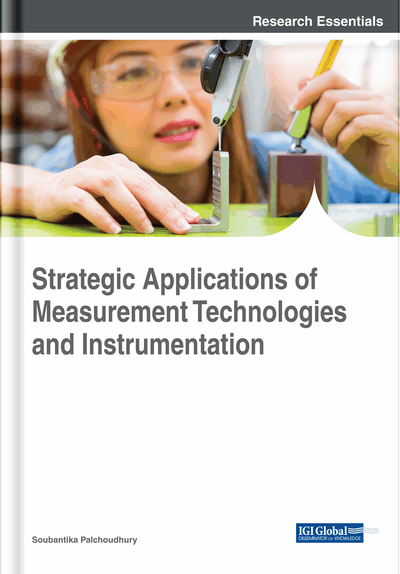# From Einstein Theories to Least Action Principle, a Relativistic Error of a Limit Case of Classical Mechanics: Matching With Measurements of Light Propagation

Alessandro Massaro (Dyrecta Lab s.r.l., Italy)
DOI: 10.4018/978-1-5225-5406-6.ch002
Available
\$37.50
No Current Special Offers

## Abstract

The authors prove that the standard least action principle implies a more general form of the same principle by which they can state generalized motion equation including the classical Euler equation as a particular case. This form is based on an observation regarding the last action principle about the limit case in the classical approach using symmetry violations. Furthermore, the well-known first integrals of the classical Euler equations become only approximate first integrals. The authors also prove a generalization of the fundamental lemma of the calculus of variation and they consider the application in electromagnetism. This chapter is an enhanced version of a published work. It proves the existence of particular relativistic error condition in classical mechanics, potentially significant on experiments of light propagation in matters. The work includes a discussion of applications potentially correlated with the found particle motion error condition.
Chapter Preview
Top

## Introduction

The least action principle is widely applicable in any field of physics and is particularly fruitful in classical mechanics (Goldstein et al., 2008). It is well known that almost all the physical equations can be derived by such a principle, so that a critical analysis about this principle is certainly useful.

The aim of this paper is to prove that its standard form implies a generalized variational principle by which we can derive generalized Euler equations: these latter are more general than the classical Euler equation and include them as a special case.

In stating our results we make use of a generalization of the fundamental lemma of the calculus of variations.

An attempt is made to interpret as a relativistic correction the difference between the generalized equations and the classical equations. Generally the new equations lead to approximate first integrals which become exact only in limiting case of the classical equations. The results of this analysis can be extended to classical field theory and to Relativistic physics and probably to Quantum mechanics and Quantum field theory (Massaro, 2011). Another application of this theory is in electron optics and, in particular, in the evaluation of the light velocity in a liquid. The chapter is an enhanced version of a work (Massaro & Massaro, 2011), which highlights the particular error condition of an analytical solution in classical mechanics theory matching with physical phenomena involving light propagation in a liquid. After an enhanced background discussion some aspect concerning the performed research and the new topics of research about relativistic error will be commented on.

## Key Terms in this Chapter

Uncertainty Principle: Any of a variety of mathematical inequalities asserting a fundamental limit to the precision with which certain pairs of physical properties of a particle, known as complementary variables, such as position x and momentum p, can be known.

Perturbation Theory: Mathematical methods for finding an approximate solution to a problem.

Particle Accelerator: Machine that uses electromagnetic fields to propel charged particles to nearly light speed and to contain them in well-defined beams.

Light Coupling: The lightwave is coupled with other electromagnetic signals.

Light Scattering: Form of scattering in which light in the form of propagating energy is scattered.

Absorption (Electromagnetic Radiation): Way in which the energy of a photon is taken up by matter, typically the electrons of an atom.

Wavenumber: Spatial frequency of a wave, either in cycles per unit distance or radians per unit distance.

## Complete Chapter List

Search this Book:
Reset